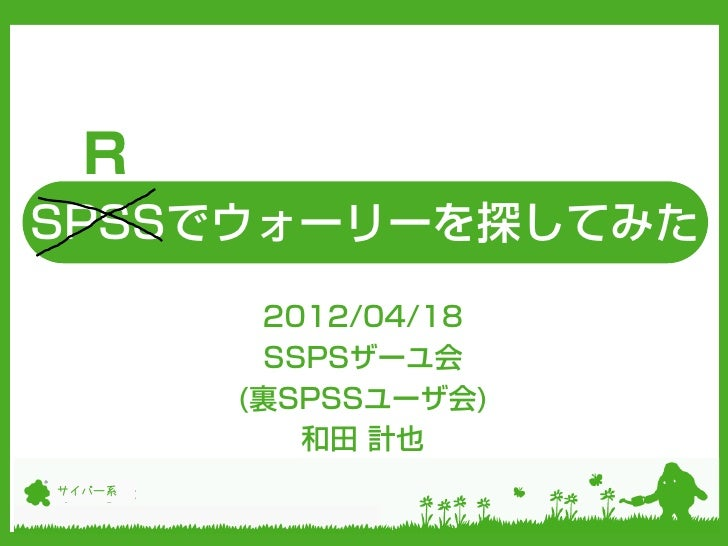Successfully reported this slideshow.Upcoming SlideShare
×

# Rでウォーリを探してみた

9,849 views

Published on

2012/4/18 SSPSザーユ会(裏SPSSユーザ会)でのLT発表資料です。主にRのEBImageパッケージを利用しています。

Published in: Education
• Full Name
Comment goes here.

Are you sure you want to Yes No• Be the first to comment

### Rでウォーリを探してみた

1. 1. RSPSSでウォーリーを探してみた 2012/04/18 SSPSザーユ会 (裏SPSSユーザ会) 和田 計也サイバー系
2. 2. サイバー系 はじめに ※この発表は個人の 見解であり、所属す る組織の公式見解で はありません。2012/04/18 1
3. 3. サイバー系 自己紹介  和田 計也（@wdkz）  静岡県袋井市出身  サイバー系企業でデータマイニングエンジニア職  創立記念日が3月18日  社長の出身地が福井県鯖江市  前職はバイオベンチャー  バイオマーカ探索してた  学生時代は枯草菌の研究2012/04/18 2
4. 4. サイバー系 ウォーリーを探せ！2012/04/18 3
5. 5. サイバー系 ウォーリーをRで探してみます！  準備  ImageMagick-6.5.9-10-Q16-windows-dll.exe  gtk2-runtime-2.16.1-2009-04-21-ash.exe (↑どちらも利用可能なバージョンがシビアなので気をつけて下さい。 最新版では動きません。ファイルはググって見つけて下さい。)  R-2.15.0の32bit版  作戦  赤色を抜き出す  ノイズ除去してから大きな塊を抽出  その塊がストライプかどうかの判定をして、もっともウォー リーっぽい物体を一つ抽出  有無を言わずそれがウォーリー2012/04/18 4
6. 6. サイバー系 事始め install.packages(c(“abline”, “biOps”)) #基本はEBImageで処理を行っていくが、画像切り出しと拡大縮小のところに biOpsを利用した。 source("http://www.bioconductor.org/biocLite.R") biocLite("EBImage") #EBImageとbiOpsのフォーマット変換に利用 source("http://rimagebook.googlecode.com/svn/installR ImageBook.R") installRImageBook() require(EBImage) require(biOps) require(RImageBook)2012/04/18 5
7. 7. サイバー系 ウォーリーのjpgファイルを読み込む wally <- readImage(file="http://www.findwaldo.com/fankit/graphics/IntlManOfLiter ature/Scenes/DepartmentStore.jpg") display(wally)2012/04/18 6
8. 8. サイバー系 赤色抽出 wally.red <- as.vector(channel(wally, "red"))*255- channel(wally,"green")*255-channel(wally, "blue")*255 wally.red[is.na(wally.red)] <- 0 wally.red[wally.red < 0] <- 0 display(wally.red)2012/04/18 7
9. 9. サイバー系 閾値を設定して2値化(強い赤みのところだけ残す) wally.red2 <- thresh(wally.red,w=3,h=3,offset=50) #二値化 display(wally.red2) #biOpsオブジェクトもつくっておく(後で使う) eb.wally <- EBI2biOps(wally.red2)2012/04/18 8
10. 10. サイバー系 ぼかしフィルタ wally.red3 <- blur(wally.red2, r=0, s=3) #ぼかし display(wally.red3)2012/04/18 9
11. 11. サイバー系 2値化 wally.red4 <- (wally.red3 > 0.15) #閾値による2値化 display(wally.red4)2012/04/18 10
12. 12. サイバー系 ノイズ除去 kern <- makeBrush(7, shape=“diamond”) #ノイズ除去用のブラシ定義 wally.red5 <- closing(opening(wally.red4,kern),kern) #ノイズ除去 display(wally.red5)2012/04/18 11
13. 13. サイバー系 塊にナンバリング wally.red5.label <- bwlabel (wally.red5) dist.res.box <- rep(-1, length=max(wally.red5.label)) #結果入れる箱 塊① for(i in 1:max(wally.red5.label)){ wally.cand <- matrix(wally.red5.label==i, 塊② nrow=nrow(wally.red5.label)) #塊のx座標、y座標を取得 y_lim <- ceiling(which(wally.cand)/nrow(wally.cand)) x_lim <- which(wally.cand)-(y_lim-1)*nrow(wally.cand) #塊周辺だけ抽出 塊③ eb.wally.crop <- imgCrop(eb.wally, x_start=min(x_lim), y_start=min(y_lim), c_width= diff(range(x_lim)), c_height= diff(range(y_lim))) 塊④ #行列にして x <- matrix(eb.wally.crop,nrow=nrow(eb.wally.crop)) 塊⑤ #塊に対しての距離を算出 dist.res.box[i] <- my.dist(x, 0.33) }2012/04/18 12
14. 14. サイバー系 (参考)距離算出関数 my.dist <- function(x, contiguity.factor=0.5){ #ストライプを20行単位で考える if(nrow(x)==20){ ストライプパターンを発見する上手な方法を x.ebi <- biOps2EBI(imagedata(x)) ご存じの方いたら教えて下さい。よろしくお願いします。 }else if(nrow(x) < 20){ #拡大の場合 x <- imgCubicScale(imagedata(x),round(20/nrow(x)*1000)/1000,round(20/nrow(x)*1000)/1000) x.ebi <- thresh(biOps2EBI(x)) }else if(nrow(x) > 20){ #縮小の場合 x <- imgMedianShrink(imagedata(x),round(20/nrow(x)*1000)/1000,round(20/nrow(x)*1000)/1000) x.ebi <- thresh(biOps2EBI(x)) } #行を集約して、一列のベクトルに変換 x2 <- apply(matrix(x.ebi,nrow=nrow(x.ebi)),2,function(y){sum(y)/length(y)}) #4つのストライプパターンを作成して tmplts <- matrix(c(rep(c(1,1,0,0,0),4), rep(c(0,1,1,0,0),4), rep(c(0,0,1,1,0),4), rep(c(0,0,0,1,1),4)),nrow=4, byrow=TRUE) #それぞれのストライプパターンとの距離を出して、最短の値を返す return(min(apply(tmplts, 1, function(y){return(sum(sqrt((x2-y)^2)) + sum(sqrt((x2[-1]-y[- length(y)])^2))*contiguity.factor + sum(sqrt((x2[-length(x2)]-y[-1])^2))*contiguity.factor)}))) }2012/04/18 13
15. 15. サイバー系 ウォーリーの塊と判定された領域を照らす w.clump <- matrix(wally.red5.label==which(dist.res.box==min(dist.res.box)), nrow=nrow(wally.red5.label)) #ウォーリーの塊のx座標、y座標を取得 y_lim <- ceiling(which(w.clump)/nrow(w.clump)) x_lim <- which(w.clump)-(y_lim-1)*nrow(w.clump) #ウォーリーの塊の中心座標 circle.center <- c(median(x_lim), median(y_lim)) #最大距離 max.radius <- max(max(abs(x_lim-circle.center)), max(abs(y_lim- circle.center))) #○のマスクを3倍増しで作成して circle.mask <- channel(drawCircle(matrix(0.5, nrow=nrow(wally), ncol=ncol(wally)), circle.center, circle.center, max.radius*3, col=1, fill=TRUE),"rgb")2012/04/18 14
16. 16. サイバー系 ウォーリーを探せた！ #元画像に上記マスクを合成 display(wally*circle.mask)2012/04/18 15
17. 17. サイバー系 おしまい 以上、 による 「ウォーリを探してみた」話でした。2012/04/18 16